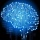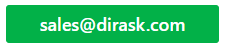Home
IT Knowledge
IT Career
Inspiration
Languages
EN

# Java - insert node as last one to double linked list (custom implementation)

1 points
Created by:Root-ssh
68500

## 1. Overview

In this post we will see how to implement algorithm to correctly add node as last one to double linked list. Below we have 2 implementations, first one with node based on Integer, second one with generic Node and usage example based on String.

• To create new Node we call constructor with next node set as null and previous node set as newly created one.
• Then we have short logic which will handle links (previous and next) between our nodes.

We can use the logic to reverse the process of adding the node as last one and implement logic for appending the node as the first one. In previous article we covered the algorithm of inserting node at the beginning of linked list (adding node as first one).

## 2. Insert node - code example

Below we have java implementation with Node based on Integer as item of double linked list with logic to insert node as last one. This implementation is without generics to have this example as simple as possible. Generic implementation of this linked list is in next part of this post.

``````package com.dirask;

Node first;
Node last;
int size;

Node tmpLast = last;
Node newNode = new Node(tmpLast, item, null);
last = newNode; // assign new node as last
if (tmpLast == null) {
first = newNode;
} else {
// take care that each node has next link
tmpLast.next = newNode;
}
size++;
}

@Override
public String toString() {
if (first != null) {
return first.printForward();
}
return "List is empty";
}

private static class Node {
Node prev;
Integer item;
Node next;

public Node(Node prev, Integer item, Node next) {
this.prev = prev;
this.item = item;
this.next = next;
}

public String printForward() {
if (next != null) {
return item + " -> " + next.printForward();
}
return String.valueOf(item);
}
}

public static void main(String[] args) {

// list: 1 -> 2 -> 3
System.out.println("list: " + list.toString());
// size: 3
System.out.println("size: " + list.size);
}
}``````

Output:

``````list: 1 -> 2 -> 3
size: 3``````

## 3. Insert node - generic implementation

Below we have java generic implementation of double linked list with add last method which will add node to our list.

``````package com.dirask;

Node<E> first;
Node<E> last;
int size;

Node<E> tmpLast = last;
Node<E> newNode = new Node<>(tmpLast, item, null);
last = newNode; // assign new node as last
if (tmpLast == null) {
first = newNode;
} else {
// take care that each node has next link
tmpLast.next = newNode;
}
size++;
}

@Override
public String toString() {
if (first != null) {
return first.printForward();
}
return "List is empty";
}

private static class Node<E> {
Node<E> prev;
E item;
Node<E> next;

public Node(Node<E> prev, E item, Node<E> next) {
this.prev = prev;
this.item = item;
this.next = next;
}

public String printForward() {
if (next != null) {
return item + " -> " + next.printForward();
}
return String.valueOf(item);
}
}

public static void main(String[] args) {

// list: 1 -> 2 -> 3
System.out.println("list: " + list.toString());
// size: 3
System.out.println("size: " + list.size);
}
}``````

Output:

``````list: 1 -> 2 -> 3
size: 3``````

## References (Harvard CS classes - Data Structures)